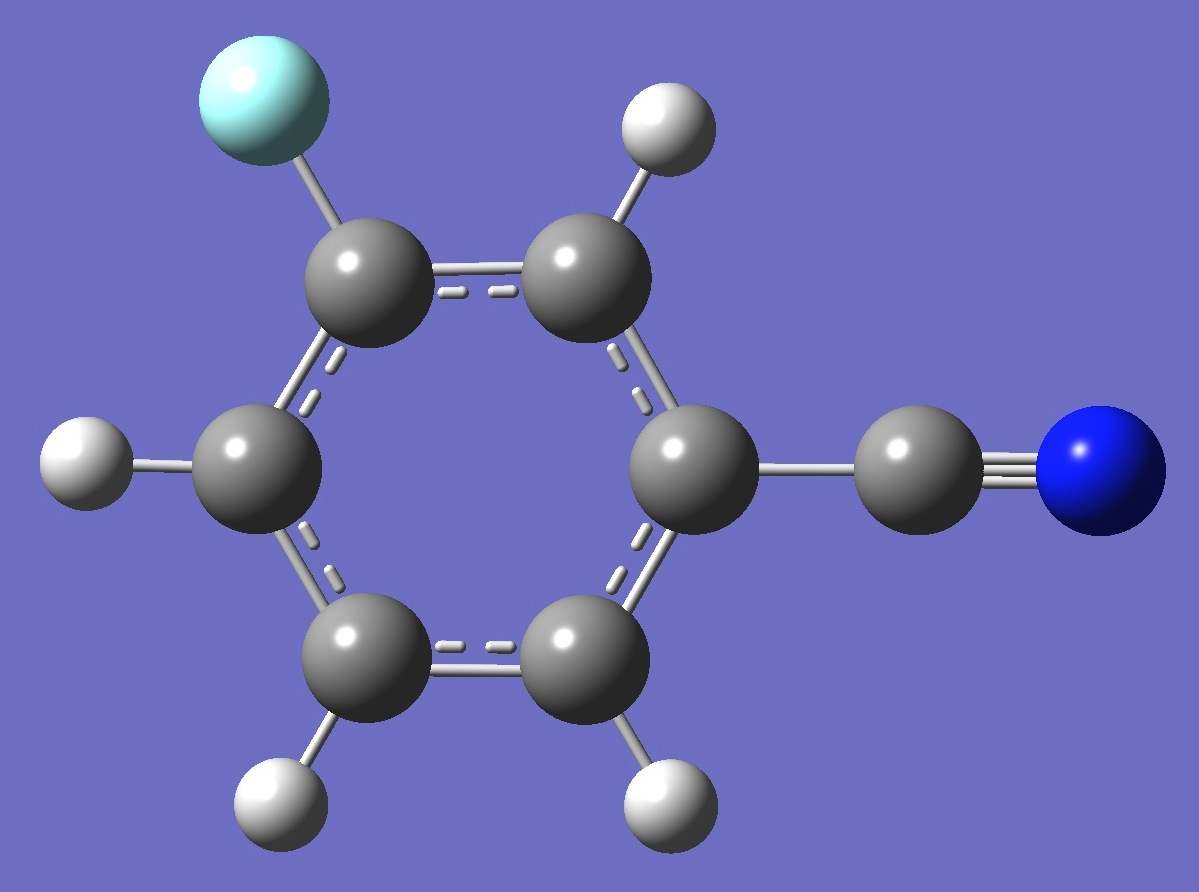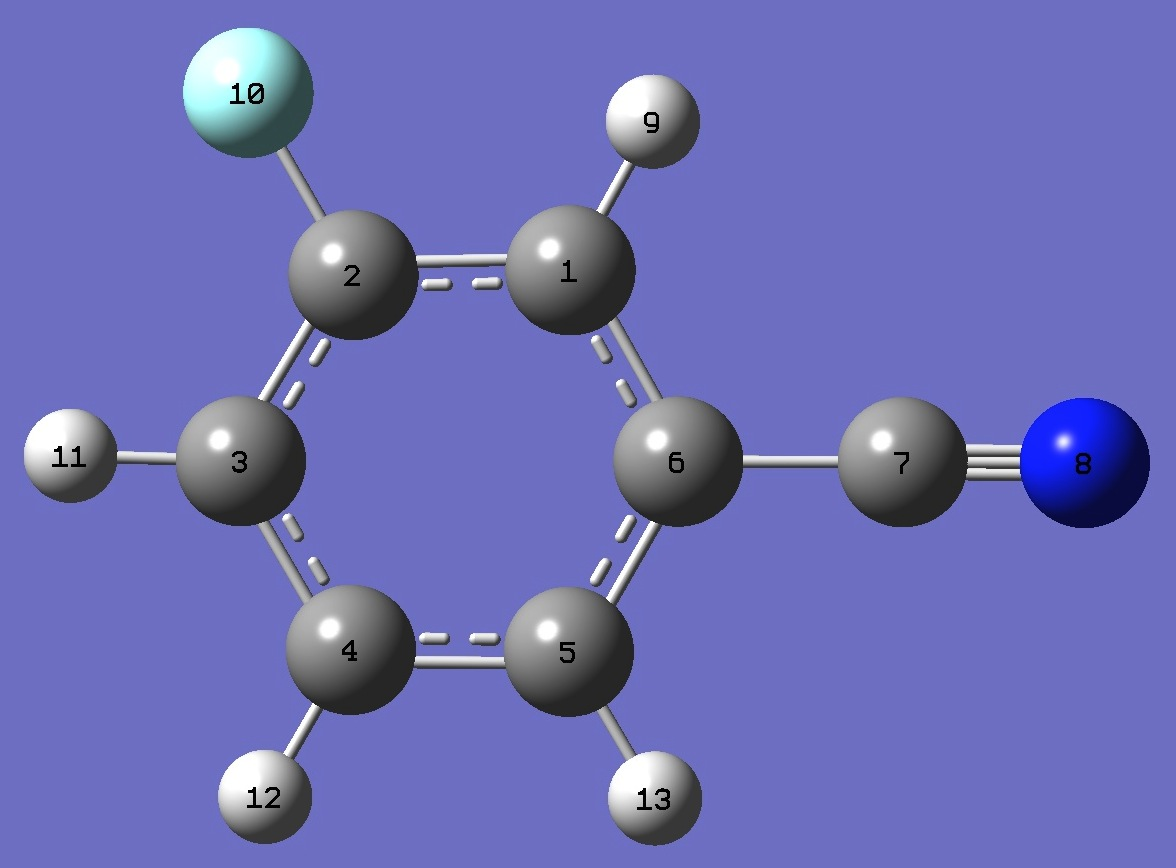m-C6H4FCNNitrogen

Nuclear Quadrupole Coupling Constants

in meta-Fluorobenzonitrile

Calculation of the nitrogen nqcc's in m-fluorobenzonitrile was made here on a molecular structure given by B3P86/6-31G(3d,3p) optimization.  These are compared with the experimental nqcc's of Böttcher and Sutter  and of Kamaee et al.  in Table 1.  Structure parameters are given in Z-Matrix format in Table 2, rotational constants and quartic centrifugal distortion constants atomic coordinates in Table 3.

In Table 1, subscripts a,b,c refer to the principal axes of the inertia tensor; x,y,z to the principal axes of the nqcc tensor.  The nqcc y-axis is chosen coincident with the inertia c-axis, these are perpendicular to the molecular plane.  Ø (degrees) is the angle between its subscripted parameters.  ETA = (Xxx - Xyy)/Xzz.

RMS is the root mean square differene between calculated and experimental diagonal nqcc's (percent of average experimental nqcc).  RSD is the calibration residual standard deviation of the B3PW91/6-311+G(df,pd) model for calculation of the efg's/nqcc's.

 Table 1. 14N nqcc's in m-Fluorobenzonitrile (MHz).  Calculation was made on the B3P86/6-31G(3d,3p) ropt structure. Calc. Expt.  Expt.  * Xaa - 3.699 - 3.682(5) - 3.6916(5) Xbb 1.712 1.696(4) 1.7017(4) Xcc 1.987 1.986(4) 1.9899(4) |Xab| 1.870 1.8608(27) RMS 0.013 (0.54 %) 0.007 (0.29 %) RSD 0.030 (1.3 %) 0.030 (1.3 %) Xxx 2.295 2.2814(16) Xyy 1.987 1.9899(4) Xzz - 4.282 - 4.2713(16) ETA - 0.0718 - 0.06825 Øz,a 17.33 17.30 Øa,CN 17.32 Øz,CN 0.01

* Calculated here from the experimental 1.5Xaa = -5.53745(68), 0.25(Xbb - Xcc) = -0.07205(16), and Xab = 1.8608(27) MHz .

 Table 2.  m-Fluorobenzonitrile.  B3P86/6-31G(3d,3p) Molecular structure parameters, ropt (Å and degrees).C  C,1,B1  C,2,B2,1,A1  C,3,B3,2,A2,1,D1,0  C,4,B4,3,A3,2,D2,0  C,1,B5,2,A4,3,D3,0  C,6,B6,1,A5,2,D4,0  N,7,B7,1,A6,6,D5,0  H,1,B8,6,A7,5,D6,0  F,2,B9,1,A8,6,D7,0  H,3,B10,2,A9,1,D8,0  H,4,B11,3,A10,2,D9,0  H,5,B12,4,A11,3,D10,0 B1=1.38196705  B2=1.38597049  B3=1.39003562  B4=1.38752294  B5=1.3978954  B6=1.42920946  B7=1.15789494  B8=1.08262073  B9=1.3385588  B10=1.0834565  B11=1.0840313  B12=1.08295712  A1=122.48884197  A2=118.62095242  A3=120.64755066  A4=118.08182062  A5=119.48246692  A6=150.23687592  A7=121.55885983  A8=118.63103727  A9=119.4537159  A10=119.5707905  A11=120.91239717  D1=0.  D2=0.  D3=0.  D4=180.  D5=180.  D6=180.  D7=180.  D8=180.  D9=180.  D10=180.

 Table 3.  m-Fluorobenzonitrile.  B3P86/6-31G(3d,3p) Rotational Constants (MHz), and B3LYP/cc-pVTZ Centrifugal Distortion Constants (kHz). Calc Expt.  A 3406.2 3388.610731(60) B 1192.0 1186.639032(12) C 883.0 878.6966531(84) Delta_J 0.0377 0.039200(60) Delta_JK 0.00467 0.01094(51) Delta_K 1.211 1.2575(53) delta_j 0.0133 0.013913(32) delta_k 0.169 0.18155(84)

 O.Böttcher and D.H.Sutter, Z.Naturforsch. 43a,47(1988).

 M.Kamaee, M.Sun, H.Luong, and J. van Wijngaarden, J.Phys.Chem. A 119(41),10279(2015).

A.Dutta, A.I.Jaman, D.K.Ghosh, and R.N.Nandi, J.Mol.Spectrosc. 118,232(1986).

Benzonitrile Pentafluorobenzonitrile

o-Fluorobenzonitrile o-Tolunitrile

p-Fluorobenzonitrile m-Tolunitrile

2,3-Difluorobenzonitrile p-Tolunitrile

Table of Contents

Molecules/Nitrogen

mC6H4FCN.html

Last Modified 28 Sept 2015